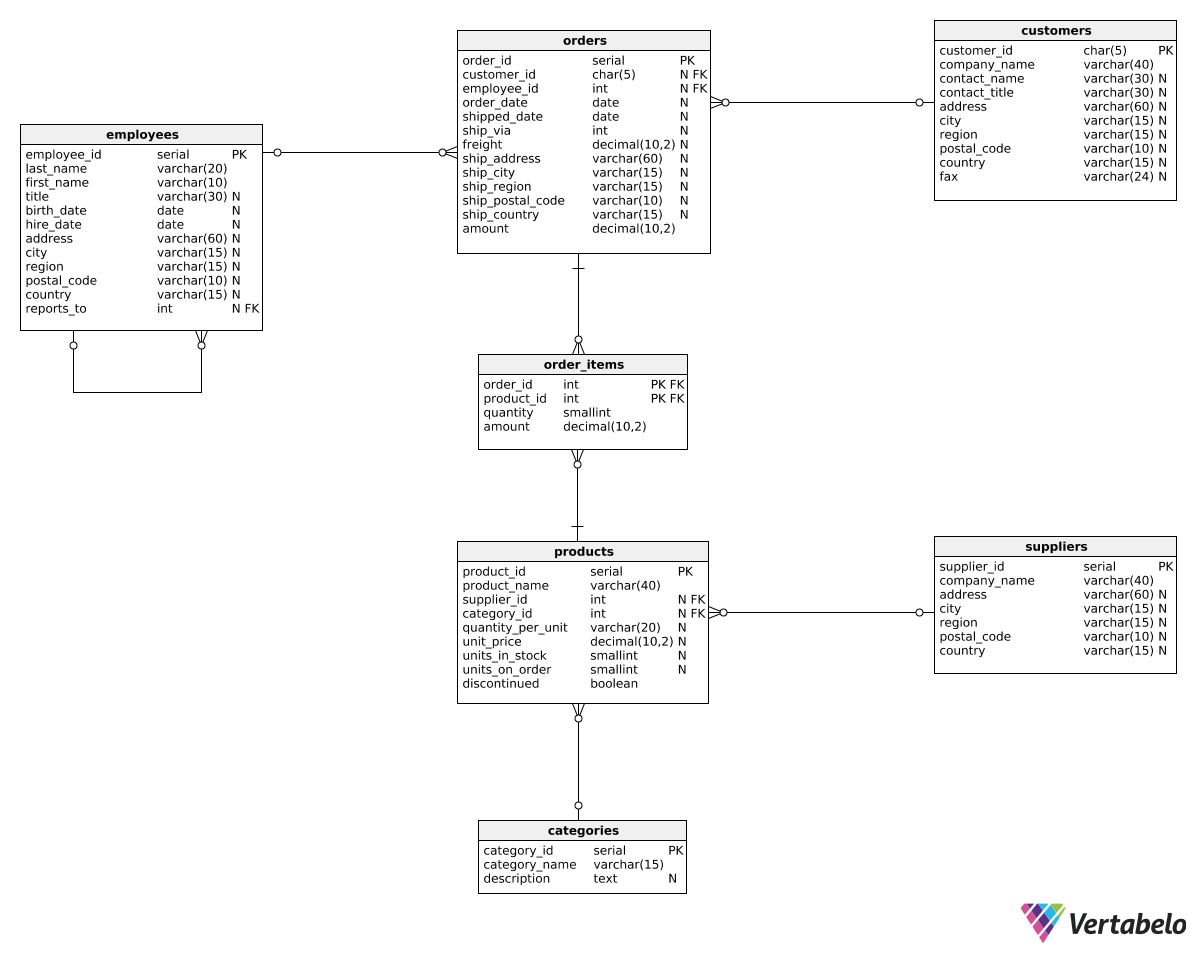Deals Of The Week - hours only!Up to 80% off on all courses and bundles.-Close
Introduction
Reports with revenue in categories
Revenue in time periods for selected categories
Summary

## Instruction

Great work! We can also create another version of this report by changing revenue values into revenue deltas. The end result should look like this:

revenue_year total_revenue_delta beverages_revenue_delta seafood_revenue_delta
2016 null null null
2017 409001.29 56005.32 47567.99
2018 -176461.34 12100.56 -22047.92

We can use the following query:

SELECT
EXTRACT(year FROM order_date) AS revenue_year,
SUM(oi.amount) - LAG(SUM(oi.amount)) OVER (ORDER BY EXTRACT(year FROM order_date)) AS total_revenue_delta,
SUM(CASE WHEN c.category_name = 'Beverages' THEN oi.amount ELSE 0.0 END)
- LAG(SUM(CASE WHEN c.category_name = 'Beverages' THEN oi.amount ELSE 0.0 END)) OVER (ORDER BY EXTRACT(year FROM order_date)) AS beverages_revenue_delta,
SUM(CASE WHEN c.category_name = 'Seafood' THEN oi.amount ELSE 0.0 END)
- LAG(SUM(CASE WHEN c.category_name = 'Seafood' THEN oi.amount ELSE 0.0 END)) OVER (ORDER BY EXTRACT(year FROM order_date)) AS seafood_revenue_delta
FROM order_items oi
JOIN orders o
ON oi.order_id = o.order_id
JOIN products p
ON p.product_id = oi.product_id
JOIN categories c
ON c.category_id = p.category_id
GROUP BY EXTRACT(year FROM order_date)
ORDER BY EXTRACT(year FROM order_date);


We now calculate each column as the sum from the current year (SUM(oi.amount)) minus the sum from the previous year (LAG(SUM(oi.amount)) OVER (ORDER BY EXTRACT(year FROM order_date))). To that end, we used the LAG() OVER() pattern we learned in the previous part.

## Exercise

Create a report similar to the one shown in the explanation. This time, however, show the revenue changes in the four quarters of 2017 for the 'Produce' and 'Confections' categories. Show the following columns: revenue_quarter, total_revenue_delta, produce_revenue_delta, and confections_revenue_delta.

### Stuck? Here's a hint!

Use a WHERE clause to filter the orders from 2017. Then, use EXTRACT(quarter FROM order_date).